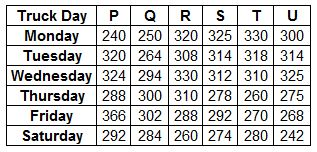### SBI Associate PO 2010 Question 145

Instructions

Study the table carefully to answer the question that follow:
Distance (in kms) travelled by six trucks on six different days of the weekQuestion 145

# If on Tuesday truck R and truck T travelled at the same speed what was the respective ratio of time taken by truck R and time taken by truck T to cover their respective distances?

Solution

If the speeds are same, the ratio of time taken is same as that of the distance travelled.
$$\frac{\text{Time taken by truck R}}{\text{Time taken by truck T}} = \frac{308}{318} = \frac{154}{159}$$